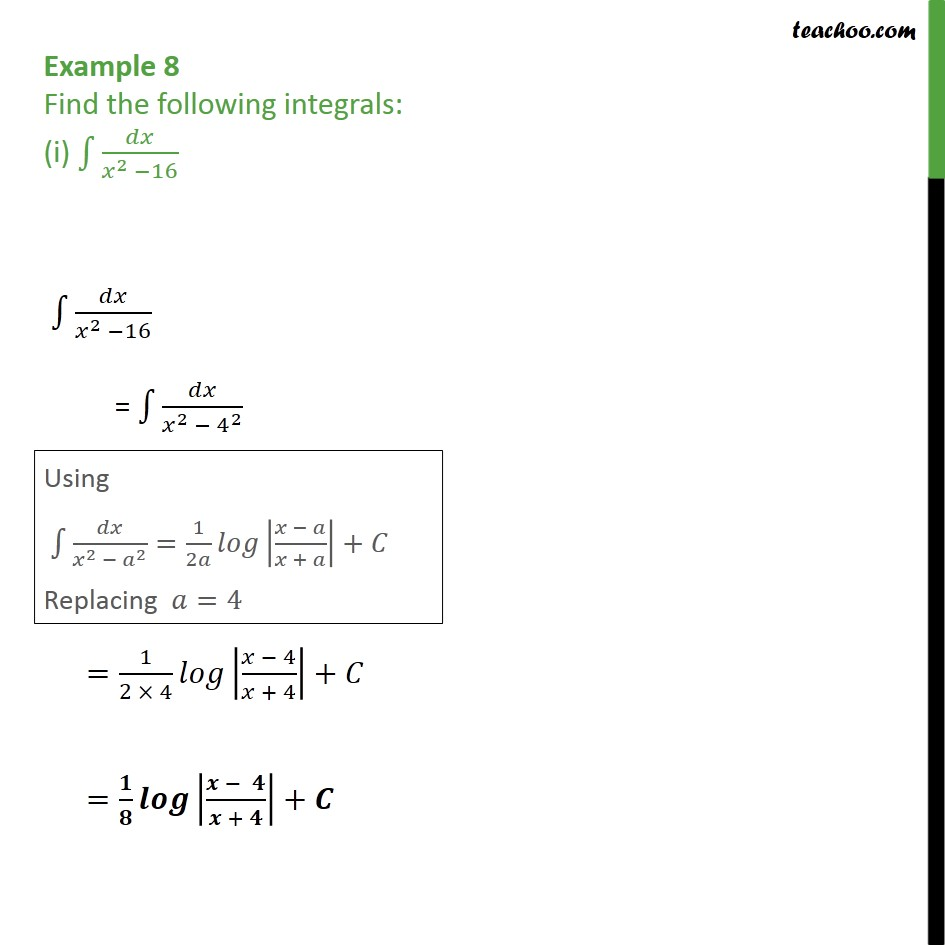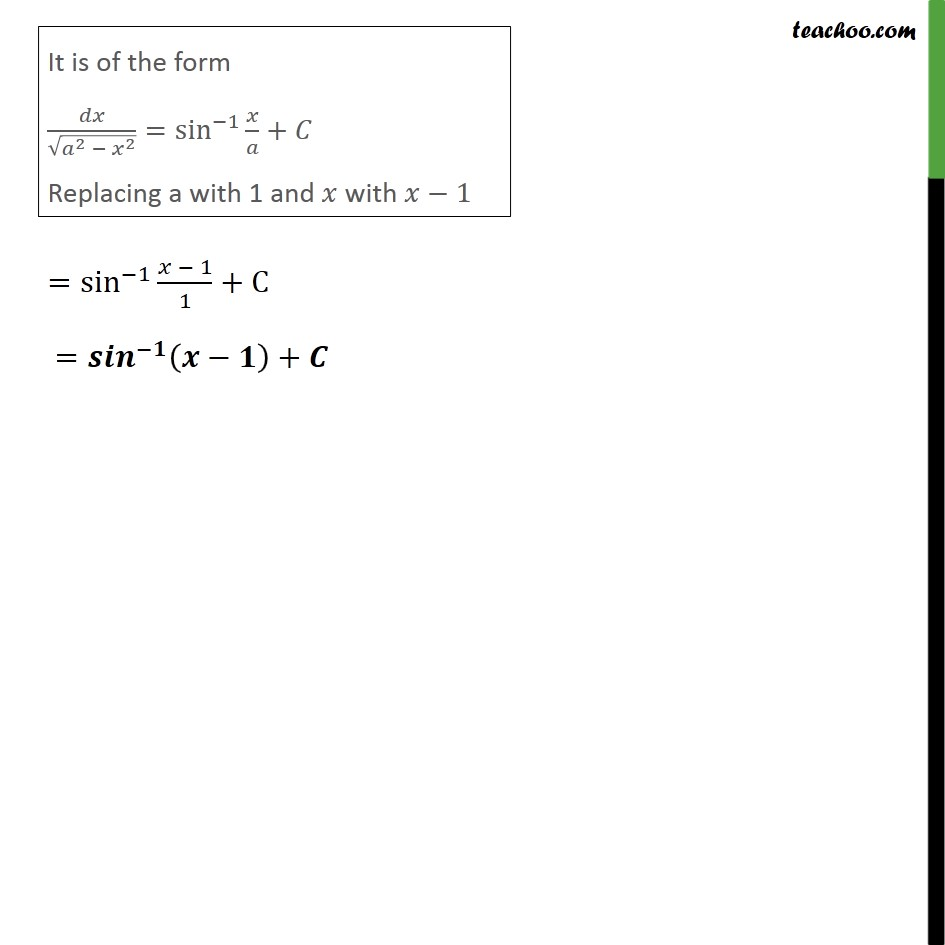Integration by specific formulaes - Formula 1

Chapter 7 Class 12 Integrals
Concept wiseLearn in your speed, with individual attention - Teachoo Maths 1-on-1 Class

### Transcript

Example 8 (i) Find the following integrals: (i) ∫ 𝑑𝑥/(𝑥^2 − 16) ∫1▒𝑑𝑥/(𝑥^2 −16) = ∫1▒𝑑𝑥/(𝑥^2 −〖 4〗^2 ) Using ∫1▒〖𝑑𝑥/(𝑥^2 − 𝑎^2 )=1/2𝑎 𝑙𝑜𝑔|(𝑥 − 𝑎)/(𝑥 + 𝑎)|+𝐶〗 Replacing 𝑎=4 =1/(2 × 4) 𝑙𝑜𝑔|(𝑥 − 4)/(𝑥 + 4)|+𝐶 =𝟏/𝟖 𝒍𝒐𝒈|(𝒙 − 𝟒)/(𝒙 + 𝟒)|+𝑪